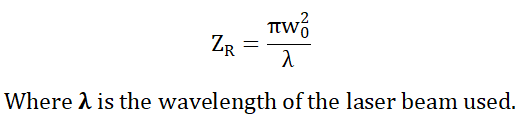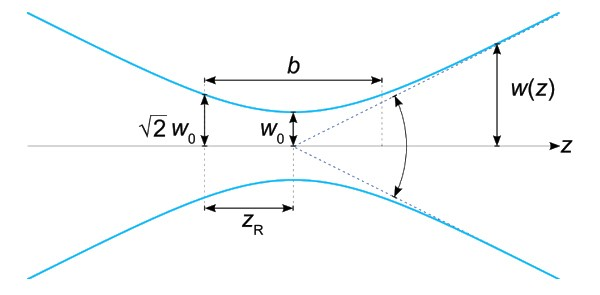# Rayleigh Length (Range) Calculator

This is an online calculator to find the rayleigh length (or rayleigh range) of a laser beam. Just enter the beam waist (or waist radius) and wavelength to get the rayleigh length value.

• mm
• nm

### Result

• Rayleigh Length (or Rayleigh Range)
m
##### Formula for Rayleigh Length (Range) CalculatorRayleigh Range (ZRis the distance from the beam waist where the beam radius has increased 1.414 times its initial value. This is the point at which the area of cross-section is doubled.If we focus a laser beam, at the focal point, theoretically the intensity goes to infinity. But this is practically not possible because a laser beam cannot have infinite intensity.

It is found that practically the laser beam cannot focus on a point, instead, there is a small beam thickness at the focal point. This smallest distance up to which a laser beam can converge is known as the beam waist (w0). It is basically the focal point of a laser beam.

So the minimum value of the beam radius is the beam waist (w0) and the beam radius goes on increasing on either side of the beam waist. The value of the beam waist determines the quality of a laser beam. It quantifies how sharply a laser beam can be focused. A low beam waist signifies a good laser quality.

Confocal Parameter (b) is the distance up to which the collimated waist region of the laser beam extends. It is twice the Rayleigh range.